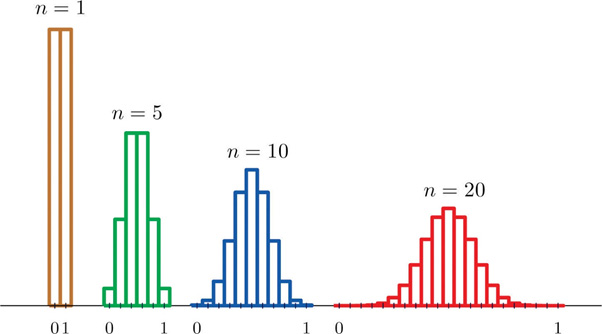# Sampling Distribution in Statistics

Sample statistics only estimate population parameters, such as the mean or standard deviation. This is because, in real-world research, only a sample of cases is selected from the population. Due to time restraints and practical issues, a researcher cannot test the total population.

Therefore, it is likely that the sample mean will be different from the (unknown) population mean. However, a researcher will never know the exact amount of sampling error, but by using a sampling distribution, they can estimate the sampling error.

## What is a sampling distribution?

A sampling distribution is a probability distribution of a statistic (such as the mean) that results from selecting an infinite number of random samples of the same size from a population.

The sampling distribution of a given population is the distribution of frequencies of a range of different outcomes that could possibly occur for a statistic of a population.

To create a sampling distribution, research must (1) select a random sample of a specific size (N) from a population, (2) calculate the chosen statistic for this sample (e.g., mean), (3) plot this statistic on a frequency distribution, and (4) repeat these steps an infinite number of times.

It is important to note that sampling distributions are theoretical, and the researcher does not select an infinite number of samples. Instead, they conduct repeated sampling from a larger population., and use the central limit theorem to build the sampling distribution.

Three different distributions are involved in building the sampling distribution.

1. The population distribution from which the random samples are selected.
2. The infinite number of random samples are selected.
3. The sampling distribution that is being created.

## The Central Limit Theorem

The central limit theorem tells us that no matter the population distribution, the sampling distribution’s shape will approach normality as the sample size (N) increases.Figure 1. Distributions of the sampling mean (Publisher: Saylor Academy).

This is useful, as the research never knows which mean in the sampling distribution is the same as the population mean, but by selecting many random samples from a population, the sample means will cluster together, allowing the research to make a very good estimate of the population mean.

Thus, as the sample size (N) increases, the sampling error will decrease.

#### Further InformationOlivia Guy-Evans

BSc (Hons), Psychology, MSc, Psychology of Education

Associate Editor for Simply Psychology

Olivia Guy-Evans is a writer and associate editor for Simply Psychology. She has previously worked in healthcare and educational sectors.Saul Mcleod, PhD

Educator, Researcher

BSc (Hons) Psychology, MRes, PhD, University of Manchester

Saul Mcleod, Ph.D., is a qualified psychology teacher with over 18 years experience of working in further and higher education.